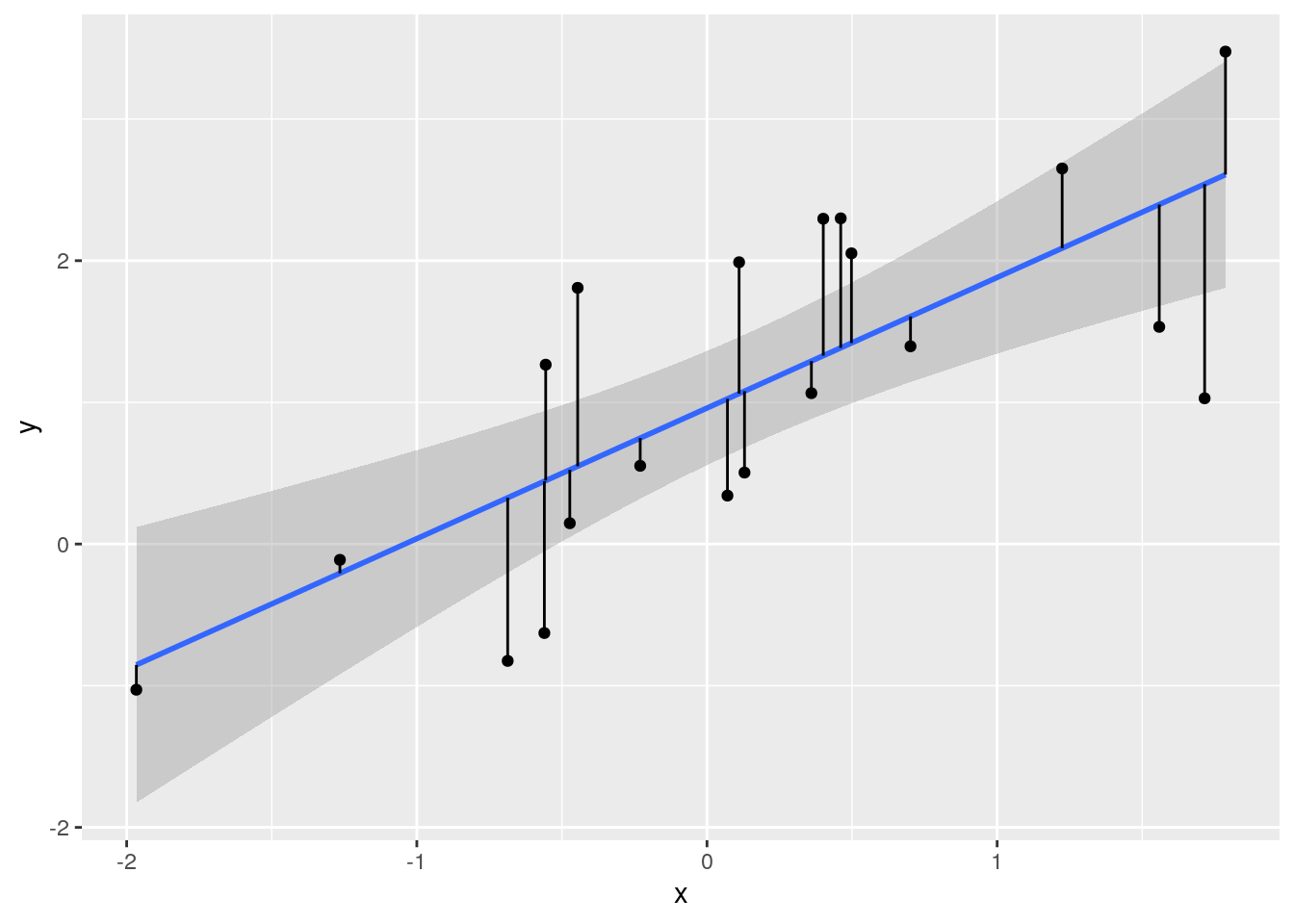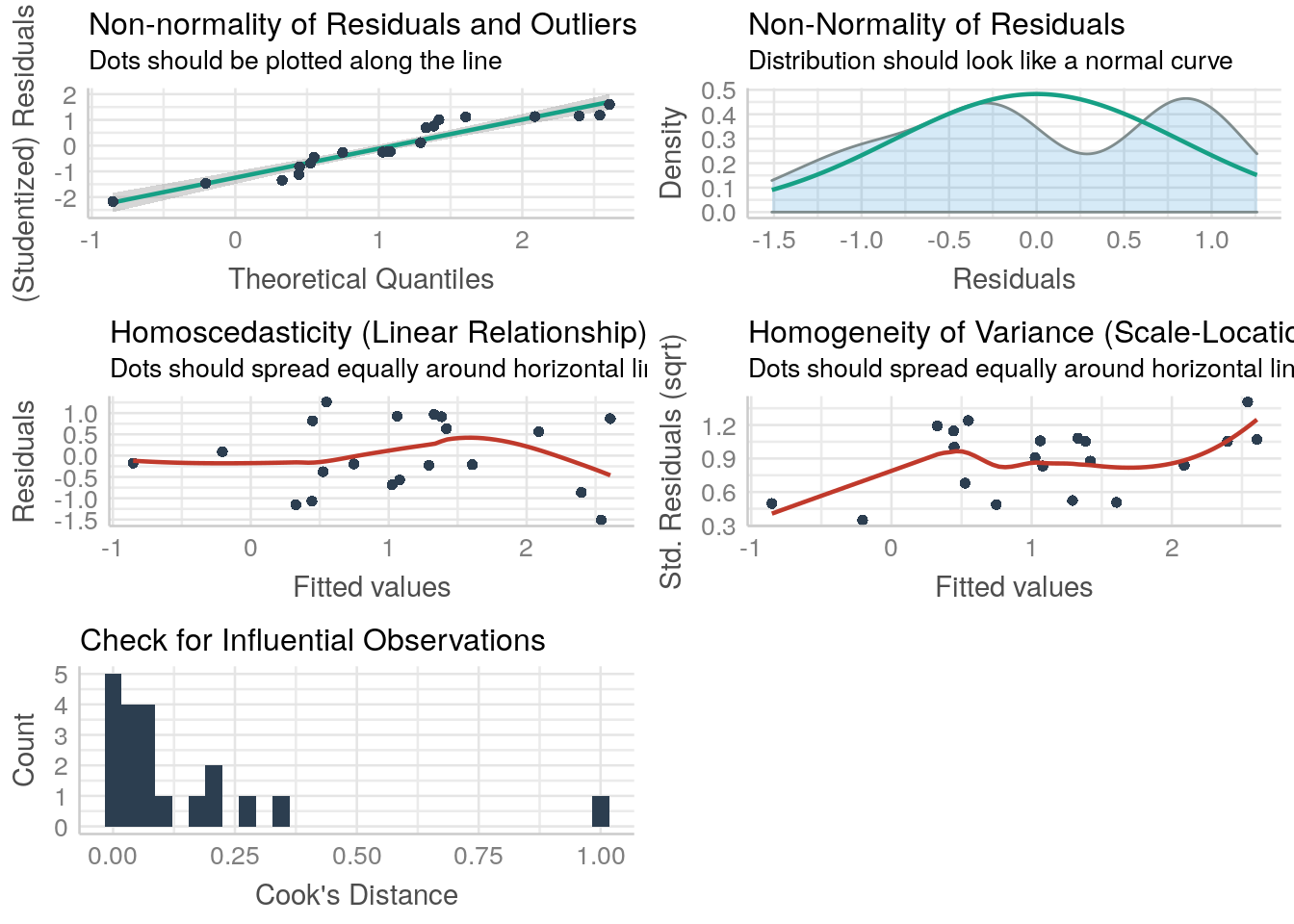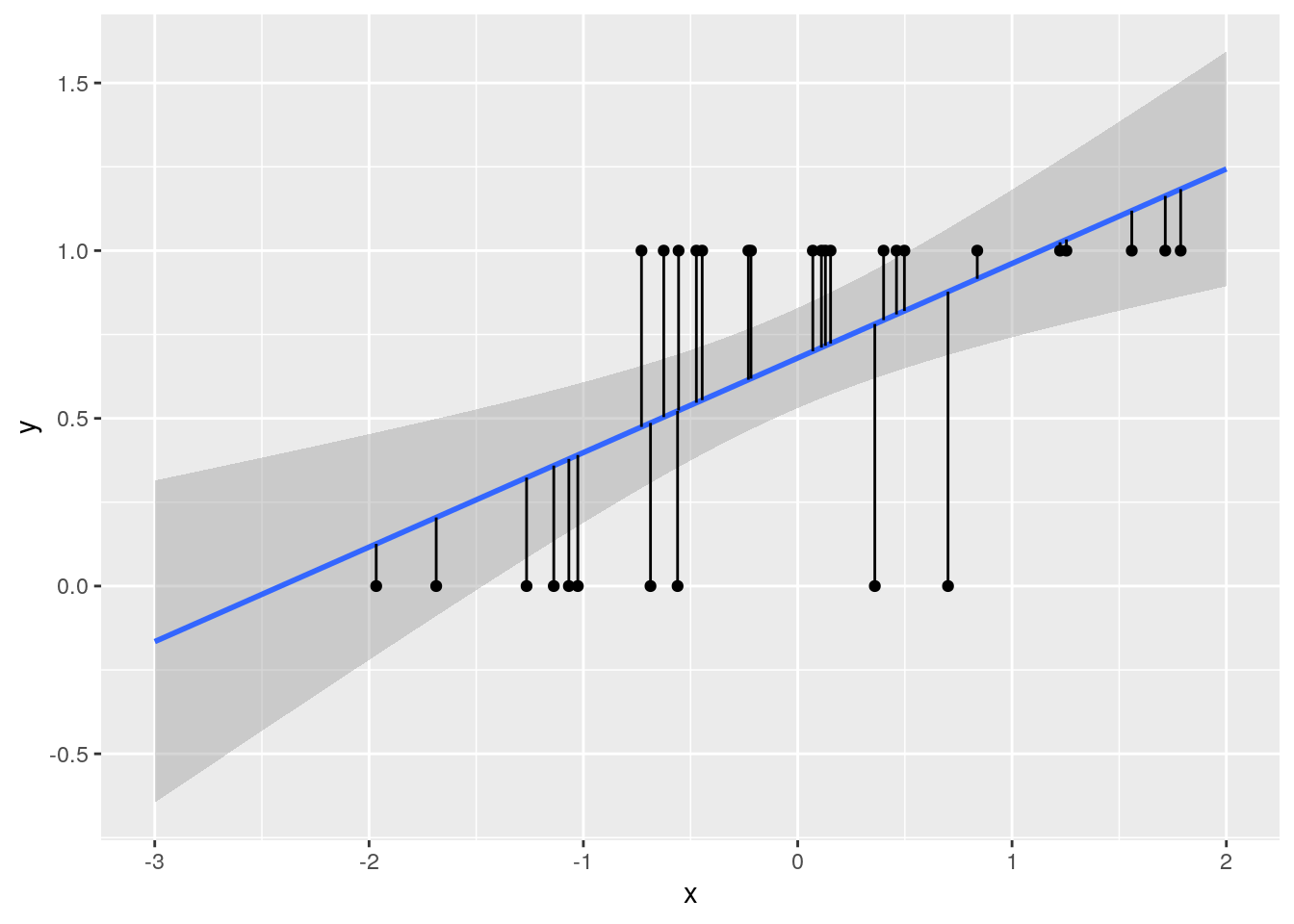Today: * Understand why linear models do not work well with some type of data, such as binary data. * Fit generalized linear models, in particular binomial (a.k.a. logistic regression) * Interpret and visualize binomial GLMs

``````download.file("https://timotheenivalis.github.io/data/survivalweight.csv",
destfile = "data/survivalweight.csv")

destfile = "data/voles.csv")``````
``````library(ggplot2)
library(performance)``````

## Failure of linear models

That’s a typical linear model (linear regression) performing okay:

``````set.seed(123)
x <- rnorm(20)
y <- 1 + x + rnorm(20)

datlinear <- data.frame(x=x, y=y)
lm0 <- lm(y~x, data = datlinear)

ggplot(datlinear, aes(x=x, y=y))+
geom_smooth(method="lm") + geom_point() +
geom_segment(aes(x=x, y=y, xend= x, yend=lm0\$fitted.values))``````
``## `geom_smooth()` using formula 'y ~ x'````check_model(lm0)``
``## Not enough model terms in the conditional part of the model to check for multicollinearity.``
``````## `geom_smooth()` using formula 'y ~ x'
## `geom_smooth()` using formula 'y ~ x'
## `geom_smooth()` using formula 'y ~ x'``````
``## `stat_bin()` using `bins = 30`. Pick better value with `binwidth`.``
``## Warning: Removed 20 rows containing missing values (geom_text_repel).``Now a model with the same structure, but fitted to binary data different data has more questionable performance:

``````set.seed(123)
x <- rnorm(30)
latent <- 1 + 2*x + rnorm(30, sd = 0.5)
y <- 1/(1+exp(-latent))
obs <- sapply(y, FUN=function(x){rbinom(1,1,x)})

datbinary <- data.frame(x=x, y=obs)
lm1 <- lm(y~x, data = datbinary)

ggplot(datbinary, aes(x=x, y=y))+
geom_smooth(method="lm", fullrange=TRUE) + geom_point() +
geom_segment(aes(x=x, y=y, xend= x, yend=lm1\$fitted.values)) +
xlim(c(-3,2))``````
``## `geom_smooth()` using formula 'y ~ x'````check_model(lm1)``
``## Not enough model terms in the conditional part of the model to check for multicollinearity.``
``````## `geom_smooth()` using formula 'y ~ x'
## `geom_smooth()` using formula 'y ~ x'
## `geom_smooth()` using formula 'y ~ x'``````
``## `stat_bin()` using `bins = 30`. Pick better value with `binwidth`.``
``## Warning: Removed 30 rows containing missing values (geom_text_repel).``